# 算法时间复杂度

+关注继续查看

算法复杂度分为时间复杂度和空间复杂度，一个好的算法应该具体执行时间短，所需空间少的特点。

随着计算机硬件和软件的提升，一个算法的执行时间是算不太精确的。只能依据统计方法对算法进行估算。我们抛开硬件和软件的因素，算法的好坏直接影响程序的运行时间。
我们看一下小例子：
int value = 0;                         // 执行了1次
for (int i = 0; i < n; i++) {       // 执行了n次
value += i;
}
这个算法执行了 1 + n 次，如果n无限大，我们可以把前边的1忽略，也就是说这个算法执行了n次
时间复杂度常用大O符号表示，这个算法的时间复杂度就是O(n).
概念： 一般情况下，算法的基本操作重复执行的次数是模块n的某一函数f(n),因此，算法的时间复杂度记做 T(n) = O(f(n))。 随着模块n的增大，算法执行的时间增长率f(n)的增长率成正比，所以f(n)越小，算法 的时间复杂度越低，算法的效率越高。
计算时间复杂度
1.去掉运行时间中的所有加法常数。
2.只保留最高阶项。
3.如果最高阶项存在且不是1，去掉与这个最高阶相乘的常数得到时间复杂度

for (int i = 0; i < n; i++) {
for (int j = i; j < n; j++) {
// do .....
}
}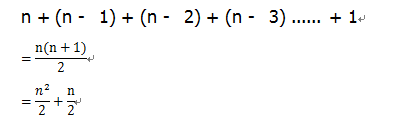1.去掉运行时间中的所有加法常数： 没有加法常数不用考虑
2.只保留最高阶项:　只保留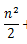3. 去掉与这个最高阶相乘的常数:  去掉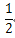只剩下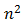最终这个算法的时间复杂度为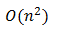for ( int i = 0; i < n; i++) {
// do .....
}
因为循环要执行n次所以时间复杂度为O(n)

 排序法 最差时间分析 平均时间复杂度 稳定度 空间复杂度 冒泡排序 O(n2) O(n2) 稳定 O(1) 快速排序 O(n2) O(n*log2n) 不稳定 O(log2n)~O(n) 选择排序 O(n2) O(n2) 稳定 O(1) 二叉树排序 O(n2) O(n*log2n) 不一顶 O(n) 插入排序 O(n2) O(n2) 稳定 O(1) 堆排序 O(n*log2n) O(n*log2n) 不稳定 O(1) 希尔排序 O O 不稳定 O(1)《算法基础：打开算法之门》一2.2 如何描述运行时间
1161 03104 01063 0477 02200 04439 01201 0javascript 一个关于时间排序的算法（一个页面多个倒计时排序）

836 0420 0979 0+关注
lpxxn

170

0

《SaaS模式云原生数据仓库应用场景实践》

《看见新力量：二》电子书